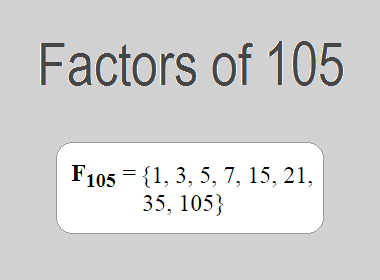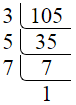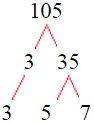# Factors of 105The factors of 105 are 1, 3, 5, 7, 15, 21, 35, and 105 i.e. F105 = {1, 3, 5, 7, 15, 21, 35, 105}. The factors of 105 are all the numbers that can divide 105 without leaving a remainder.

We can check if these numbers are factors of 105 by dividing 105 by each of them. If the result is a whole number, then the number is a factor of 105. Let's do this for each of the numbers listed above:

·        1 is a factor of 105 because 105 divided by 1 is 105.

·        3 is a factor of 105 because 105 divided by 3 is 35.

·        5 is a factor of 105 because 105 divided by 5 is 21.

·        7 is a factor of 105 because 105 divided by 7 is 15.

·        15 is a factor of 105 because 105 divided by 15 is 7.

·        21 is a factor of 105 because 105 divided by 21 is 5.

·        35 is a factor of 105 because 105 divided by 35 is 3.

·        105 is a factor of 105 because 105 divided by 105 is 1.

## How to Find Factors of 105?

1 and the number itself are the factors of every number. So, 1 and 105 are two factors of 105. To find the other factors of 105, we can start by dividing 105 by the numbers between 1 and 105. If we divide 105 by 2, we get a remainder of 1. Therefore, 2 is not a factor of 105. If we divide 105 by 3, we get a remainder of 0. Therefore, 3 is a factor of 105.

Next, we can check if 4 is a factor of 105. If we divide 105 by 4, we get a remainder of 1. Therefore, 4 is not a factor of 105. We can continue this process for all the possible factors of 105.

Through this process, we can find that the factors of 105 are 1, 3, 5, 7, 15, 21, 35, and 105. These are the only numbers that can divide 105 without leaving a remainder.

********************

********************

## Properties of the Factors of 105

The factors of 105 have some interesting properties. One of the properties is that the sum of the factors of 105 is equal to 192. We can see this by adding all the factors of 105 together:

1 + 3 + 5 + 7 + 15 + 21 + 35 + 105 = 192

Another property of the factors of 105 is that they are all odd numbers. This is because 105 is an odd number, and any even number cannot divide an odd number without leaving a remainder.

Another property of the factors of 105 is that the prime factors of 105 are 3, 5, and 7 only.

## Applications of the Factors of 105

The factors of 105 have several applications in mathematics. One of the applications is in finding the highest common factor (HCF) of two or more numbers. The HCF is the largest factor that two or more numbers have in common. For example, to find the HCF of 105 and 75, we need to find the factors of both numbers and identify the largest factor they have in common. The factors of 105 are 1, 3, 5, 7, 15, 21, 35, and 105. The factors of 75 are 1, 3, 5, 15, 25, and 75. The largest factor that they have in common is 15. Therefore, the HCF of 105 and 75 is 15.

Another application of the factors of 105 is in prime factorization. Prime factorization is the process of expressing a number as the product of its prime factors. The prime factors of 105 are 3, 5, and 7 since these are the only prime numbers that can divide 105 without leaving a remainder. Therefore, we can express 105 as:

105 = 3 × 5 × 7

We can do prime factorization by division and factor tree method also. Here is the prime factorization of 105 by division method,105 = 3 × 5 × 7

Here is the prime factorization of 105 by the factor tree method,105 = 3 × 5 × 7

## Conclusion

The factors of 105 are the numbers that can divide 105 without leaving a remainder. The factors of 105 are 1, 3, 5, 7, 15, 21, 35, and 105. The factors of 105 have some interesting properties, such as being odd numbers and having a sum of 192. The factors of 105 have several applications in mathematics, such as finding the highest common factor and prime factorization.